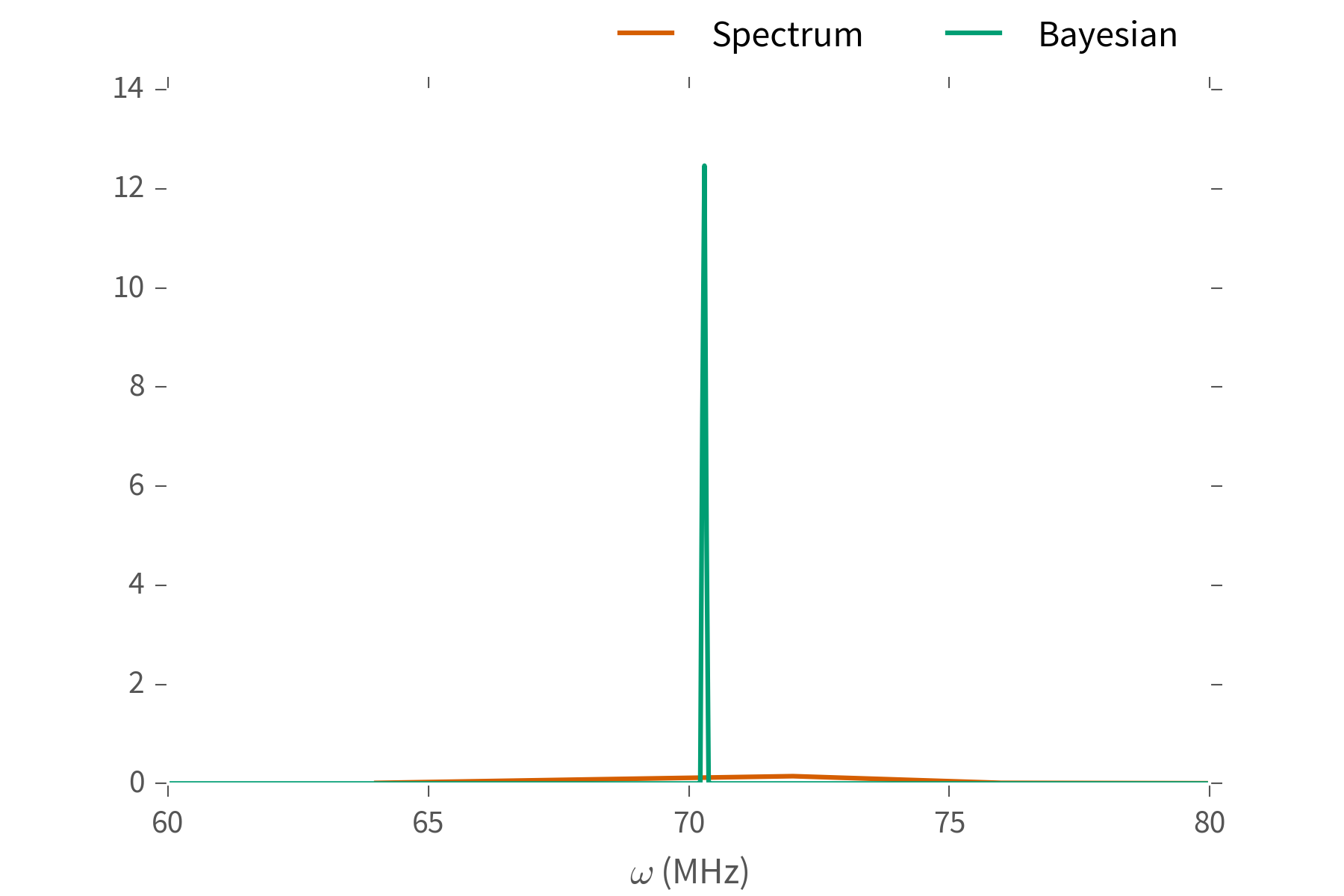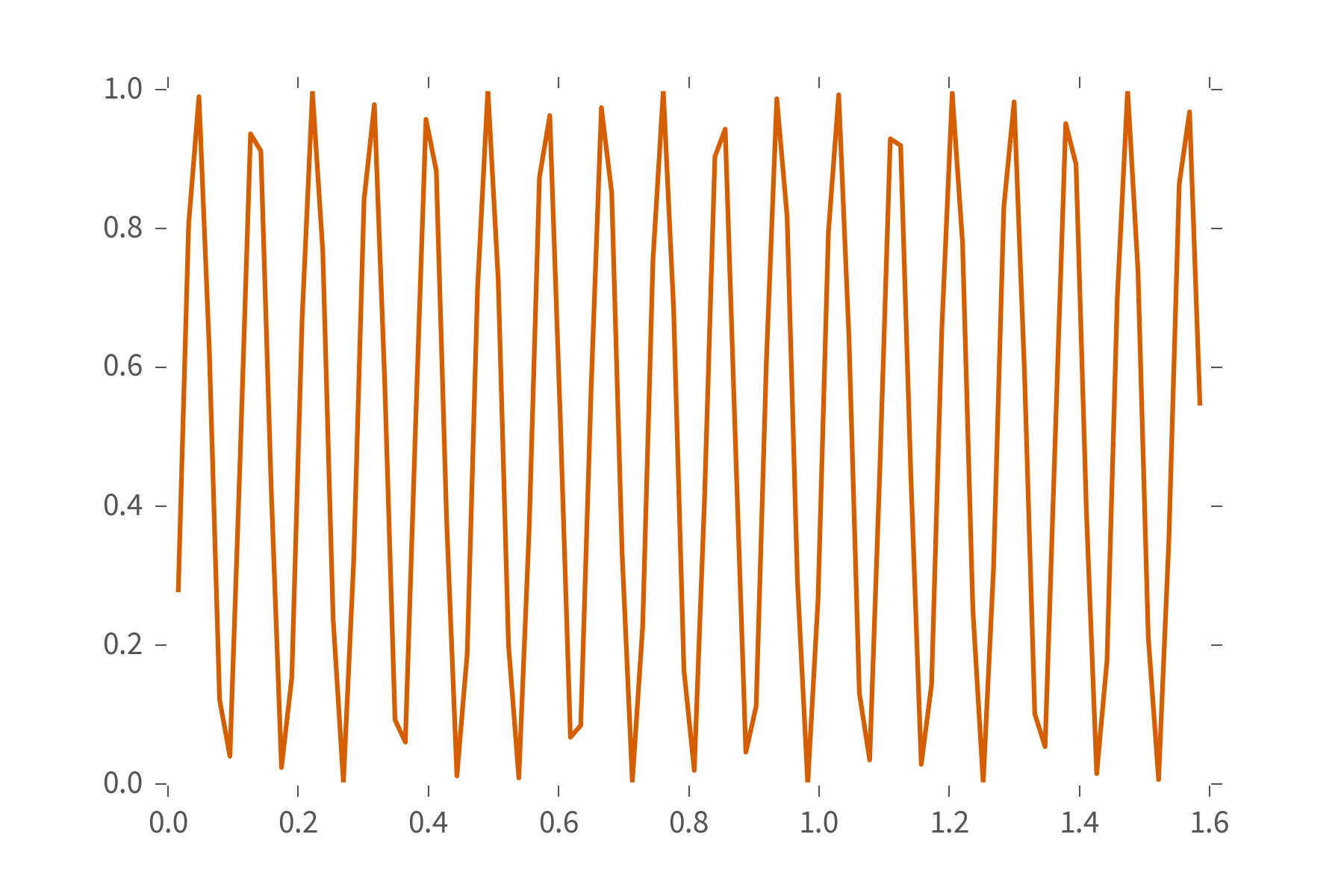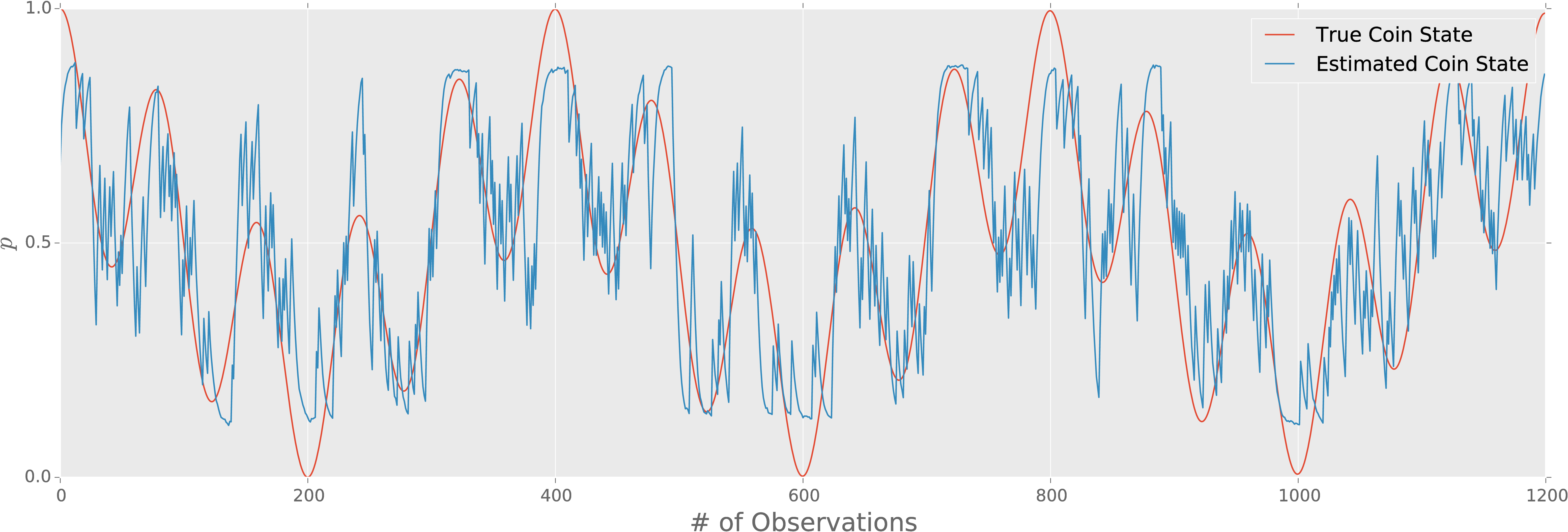# QInfer: Bayesian inference for quantum information

10/s87, 10/bh6w $\newcommand{\ee}{\mathrm{e}} \newcommand{\ii}{\mathrm{i}} \newcommand{\dd}{\mathrm{d}} \newcommand{\id}{𝟙} \newcommand{\TT}{\mathrm{T}} \newcommand{\defeq}{\mathrel{:=}} \newcommand{\Tr}{\operatorname{Tr}} \newcommand{\Var}{\operatorname{Var}} \newcommand{\Cov}{\operatorname{Cov}} \newcommand{\rank}{\operatorname{rank}} \newcommand{\expect}{\mathbb{E}} \newcommand{\sket}{|#1\rangle\negthinspace\rangle} \newcommand{\sbraket}{\langle\negthinspace\langle#1\rangle\negthinspace\rangle} \newcommand{\Gini}{\operatorname{Ginibre}} \newcommand{\supp}{\operatorname{supp}} \newcommand{\ket}{\left|#1\right\rangle} \newcommand{\bra}{\left\langle#1\right|} \newcommand{\braket}{\left\langle#1\right\rangle}$

joint work with Christopher Ferrie

contributions from:
Steven Casagrande, Ian Hincks, Jonathan Gross, Michal Kononenko, Thomas Alexander, and Yuval Sanders

Characterization plays a number of different roles in quantum information experiments.

Calibration
(Rabi/Ramsey/phase est., crosstalk learning)
Diagnosis and Debugging
(Tomography)
Verification and Validation
(RB, quantum Hamiltonian learning)

All of these are examples of parameter estimation.

## Parameter Estimation ## Given data $D$, and a model $\vec{x}$, what should we estimate $\vec{x}$ as? - Rabi/Ramsey: $\vec{x} = (\omega)$ - Crosstalk/Hamiltonian learning: $\vec{x} = \operatorname{vec}(H)$ - Tomography: $\vec{x} = \operatorname{vec}(\rho)$ --- From an experimental perspective, parameter estimation isn't the point, but a tool to get things done.
### **Example**: Ramsey Estimation ### Suppose $H = \omega \sigma_z / 2$ for some unknown $\omega$. To learn $\omega$: - Prepare $\ket{+} \propto \ket{0} + \ket{1}$, measure “click” w/ pr.: $\|\bra{+} \ee^{\ii \omega t \sigma_z / 2} \ket{+}\|^2 = \cos^2(\omega t / 2)$. - Repeat for many “shots” to estimate click pr. - Repeat for many times to estimate signal.
You'll get something that looks a bit like this: ![](figures/rabi-example-signal.png)
What's $\omega$? Fourier transform and look at the peak. ![](figures/rabi-example-spectrum.png)

We can do better.
Ex.: Sergeevich et al. 10/c4vv95, Ferrie et al. 10/tfx, Hall and Wiseman 10/bh6vOur goal is to make useful tools for parameter estimation that work in practice, in a statistically-principled fashion.

Make it easier to get experiments done:

• Reduce data collection costs
• Provide accurate estimates
• Support reproducible research practices.

Our theoretical basis will be

### Bayesian Inference with Particle Filtering.

Represent our beliefs about the model by a set of hypotheses $\{\vec{x}_i\}$, along with their weights $\{w_i\}$.

\begin{align*} \text{Estimate: } \hat{x} & = \sum_i w_i \vec{x}_i \\ \text{Update: } w_i' & \propto w_i \times \Pr(D | \vec{x}_i) \end{align*}

Numerical stability is provided by resampling:

• Contract hypotheses towards center of mass
• Convolve with Gaussian

Preserves estimates and errors of hypotheses $\{\vec{x}\}$, while restoring stability of the approximation.

- Statistically principled: approximates Bayesian posteriors. - Very general approach. - Provides rich error reporting, model selection and other diagnostics.

### >>> import qinfer

Implements particle filtering, with support for common quantum information models:

• Hamiltonian learning / phase estimation
• Randomized benchmarking
• State and process tomography
### Scientific Software is for People, not Computers ### Thus, **QInfer** is: - **Accessible**: written in Python, usable from Python, Julia, MATLAB. - **Open source**: modifiable and reproducible. - **Portable**: Windows/Linux/OS X. - **Legible**: [mostly] well-documented (guide & examples).
### Installing **QInfer** ### bash $pip install qinfer  Works on Python 2.7, 3.3, 3.4, and 3.5 with the Anaconda Distribution. ### Using **QInfer**: Simple Estimation ### python import numpy as np import qinfer as qi # Make the data... true_omega = 70.3 n_shots = 100 ts = np.pi * np.arange(1, 101) / (2 * 100.0); signal = np.sin(true_omega * ts / 2) ** 2; counts = np.random.binomial(n=n_shots, p=ideal_signal) # ...and then process it. data = np.column_stack([counts, ts, n_shots * np.ones(len(ts))]) est_mean, est_cov = qi.simple_est_prec(data, freq_min=0, freq_max=100)  ### Using **QInfer** from MATLAB 2016a ### matlab % Make the data... true_omega = 70.3; n_shots = 400; ts = pi * (1:1:100) / (2 * 100); signal = sin(true_omega * ts / 2) .^ 2; counts = binornd(n_shots, signal); % ... and then process it. setenv MKL_NUM_THREADS 1 data = py.numpy.column_stack({counts ts ... n_shots * ones(1, size(ts, 2))}); est = py.qinfer.simple_est_prec(data, ... pyargs('freq_min', 0, 'freq_max', 100));  ### Using **QInfer** from Julia ### julia @pyimport numpy as np @pyimport qinfer as qi # Make the data... true_omega = 70.3 n_shots = 100 ts = pi * (1:1:100) / (2 * 100) signal = sin(true_omega * ts / 2) .^ 2 counts = map(p -> rand(Binomial(n_shots, p)), signal); # ...and then process it. data = [counts'; ts'; n_shots * ones(length(ts))']' est_mean, est_cov = qi.simple_est_prec(data, freq_min=0, freq_max=100)  ### Breaking it Down ### **QInfer** is built up of several main components: - Model: Specifies a model for what parameters describe an experiment. - Distribution: Specifies what is known about those parameters at the start. - SMCUpdater: Uses sequential Monte Carlo to update knowledge based on data. - Heuristic: Selects new experiments to perform. ### **QInfer** Concepts: Models ### Parameter esimation problems are specified as **models**, defining parameters of interest, what data looks like, etc. python >>> SimplePrecessionModel() >>> BinomialModel(RandomizedBenchmarkingModel()) >>> BinomialModel(TomographyModel(basis))  ### **QInfer** Concepts: Models ### Models expose two very important functionalities: - simulate_experiment: Simulates data$d$from an experiment$e$, according to a set of model parameters$\vec{x}$. - likelihood: Returns the probability$\Pr(d | \vec{x}; e)$of observing$d$in an experiment$e$given model parameters$\vec{x}$.  outcomes = np.array() modelparams = np.array([w]) expparams = ts L = SimplePrecessionModel().likelihood( outcomes, modelparams, expparams ) plt.plot(ts, L[0, 0, :])### **QInfer** Concepts: Distributions ### python >>> UniformDistribution([0, omega_max])  Represents that$\omega \in [0, \omega_\max]$. Distributions can also be combined in different ways: python >>> ProductDistribution( ... NormalDistribution([0.9, 0.1 ** 2]), ... UniformDistribution([0, 1]), ... ConstantDistribution(0) ... )  ### Using **QInfer**: Updating ### Typically, once you have a model and a prior, learning parameters then proceeds by looping over data: python >>> updater = SMCUpdater(model, n_particles, prior) >>> for idx in range(n_measurements): ... experiment = # select the next experiment ... datum = # make a measurement ... updater.update(datum, experiment) >>> est = updater.est_mean()  ### Using **QInfer**: Updating ### The updated distribution provides estimates, error bars, and plots. python >>> mean, cov, extra = qi.simple_est_rb( ... data, p_min=0.8, return_all=True ... ) >>> print(mean, "±", np.sqrt(cov)[0, 0]) 0.991188359708 ± 0.0012933975599 >>> print(np.sqrt(np.diag(cov))) >>> extra['updater'].plot_posterior_marginal(idx_param=0) >>> extra['updater'].plot_covariance(corr=True)  ### **QInfer** Concepts: Heuristics ### *Heuristics* can be used to design measurements. For example,$t_k = ab^k$is optimal for non-adaptive Rabi/Ramsey/phase estimation.  >>> heuristic = ExpSparseHeuristic(scale=a, base=b)  **QInfer** implements heuristics as functions which provide new experiments. ### Updater Loops Revisited ### For instance, using a heuristic heuristic_class and simulating data, we can flesh out the updater loop. python >>> updater = SMCUpdater(model, n_particles, prior) >>> heuristic = heuristic_class(updater) >>> for idx in range(n_measurements): ... experiment = heuristic() ... datum = model.simulate_experiment(true_model, experiment) ... updater.update(datum, experiment) >>> est = updater.est_mean()  ### Tomography Updater Loop ### python from qutip import * I, X, Y, Z = qeye(2), sigmax(), sigmay(), sigmaz() basis = pauli_basis(1) prior = GinibreReditDistribution(basis) model = BinomialModel(qi.tomography.TomographyModel(basis)) updater = SMCUpdater(model, 2000, prior) heuristic = RandomPauliHeuristic(updater, other_fields={'n_meas': 40} ) for idx_exp in xrange(50): experiment = heuristic() datum = model.simulate_experiment(true_state, experiment) updater.update(datum, experiment)  ### **QInfer** Concepts: Performance Testing ### In both of these examples, we assumed that the true model was known. This lets us quickly assess how well **QInfer** works for a given model. python >>> performance = perf_test_multiple( ... n_trials=400, ... model=BinomialModel(SimplePrecessionModel()), ... n_particles=2000, ... prior=UniformDistribution([0, 1]), ... n_exp=200, ... heuristic_class=partial( ... ExpSparseHeuristic, other_fields={'n_meas': 40} ... ) ... )  ### Diffusive Models ### **QInfer** also supports time-dependent parameter estimation by adding an update rule to hypothesis positions as well as weights: $$\vec{x}(t_{k + 1}) - \vec{x}(t_k) \sim \mathcal{N}(0, \sigma^2).$$ Diffusive estimation can still work even if the underlying trajectory is deterministic.For example, suppose a coin bias evolves as$\Pr(p) = \frac12 (\cos^2(\omega_1 t / 2) + \cos^2(\omega_2 t) / 2))\$.

Our hope is that **QInfer** will thus be a useful tool for theory and experiment alike. --- - Source: https://github.com/QInfer/python-qinfer - Try it out online: https://goo.gl/zWt9Du *Version 1.0 coming soon.*